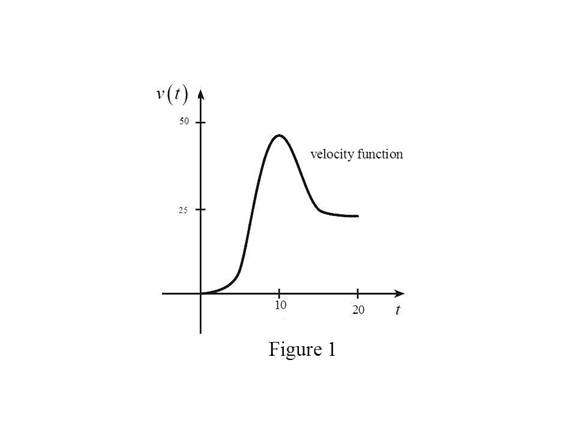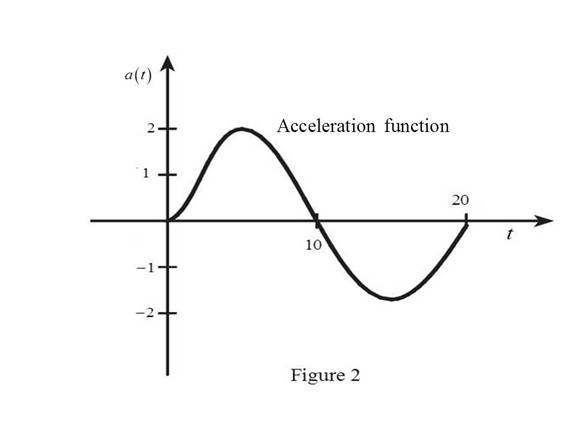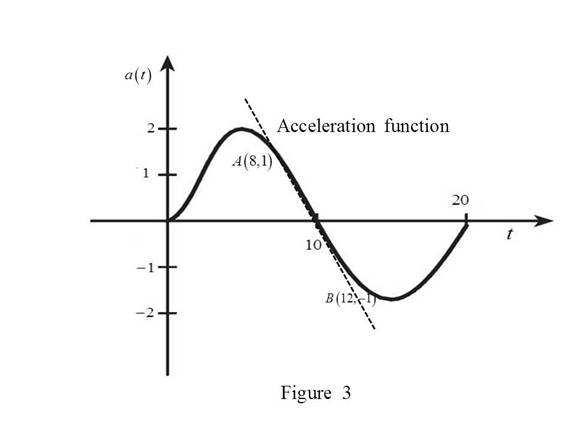# The acceleration at t = 10 seconds by using the graph of velocity and acceleration of the car.### Single Variable Calculus: Concepts...

4th Edition
James Stewart
Publisher: Cengage Learning
ISBN: 9781337687805### Single Variable Calculus: Concepts...

4th Edition
James Stewart
Publisher: Cengage Learning
ISBN: 9781337687805

#### Solutions

Chapter 2.7, Problem 48E

(a)

To determine

## To find: The acceleration at t=10 seconds by using the graph of velocity and acceleration of the car.

Expert Solution

At t=10 seconds, the acceleration is 0 ft s-2.

### Explanation of Solution

The graph of the position function is given with s in feet and t in seconds.

From the given graph, it is observed that the slope of the position function is always positive for t>0. So, the velocity curve always will have a positive functional value.

The slope of the curve increases very slowly initially, then just before t=10.

The slope of the curve increases very rapidly and then the increase in slope decreases just after t=10.

After the time just before t=20, the slope decreases rapidly.

Graph:

Use the above information and trace the graph of velocity function as shown below in Figure 1.From Figure 1, it is observed that velocity contains the horizontal tangent at one point.

Let the point be A.

Note that, the value of the derivative will be zero at the point where the function has the horizontal tangent.

Thus, the graph of acceleration will be zero at the points A.

At t=0, the slope of velocity function is zero. After sometime, the slope increases and then decreases after t=0.

At t=20, the slope of velocity function is almost horizontal.

Use the above information and trace the graph of acceleration function as shown below in Figure 2.From Figure 2, it is observed that the acceleration is positive in the first half and negative in the second half.

From the Figure 2, at t=10 seconds, the acceleration is 0 ft s-2.

(b)

To determine

### To calculate: The jerk at t=10 using the acceleration curve from part (a) and write the units.

Expert Solution

The jerk at t=10 is 0.5 and the unit of jerk is ft s-3.

### Explanation of Solution

Estimation:

Draw the tangent at t=10 as shown in Figure 3Calculation:

The jerk at t=10 is the derivative of acceleration function at t=10.

From the Figure 3, take two points in the vicinity of t=10.

Let these two point be A and B.

Slope of the curve at t=10 is equal to the slope of the line joining A to B.

m=11128=24=12

Thus, m=12.

Therefore, the jerk at t=10 is 0.5 and the unit of jerk is ft s-3.

### Have a homework question?

Subscribe to bartleby learn! Ask subject matter experts 30 homework questions each month. Plus, you’ll have access to millions of step-by-step textbook answers!